Courses

# Introduction to Motion Class 11 Notes | EduRev

## JEE : Introduction to Motion Class 11 Notes | EduRev

The document Introduction to Motion Class 11 Notes | EduRev is a part of the JEE Course Physics Class 11.
All you need of JEE at this link: JEE

MOTION IN A STRAIGHT LINE

Motion
If an object changes its position with respect to its surroundings with time, then it is called in motion.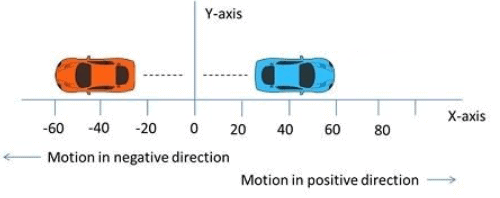Fig: Straight line motion

Rest
If an object does not change its position with respect to its surroundings with time, then it is called at rest.

[Rest and motion are relative states. It means an object which is at rest in one frame of reference can be in motion in another frame of reference at the same time.]

Point Mass Object
An object can be considered as a point mass object, if the distance travelled by it in motion is very large in comparison to its dimensions.

Types of Motion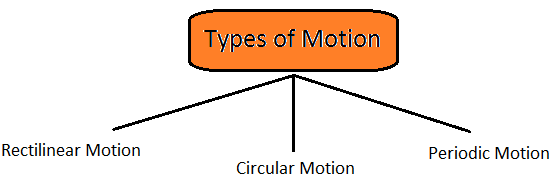1. One Dimensional Motion
If only one out of three coordinates specifying the position of the object changes with respect to time, then the motion is called one dimensional motion.

For instance, motion of a block in a straight line motion of a train along a straight track a man walking on a level and narrow road and object falling under gravity etc.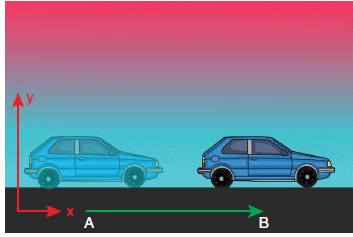Fig: One Dimensional Motion

2. Two Dimensional Motion
If only two out of three coordinates specifying the position of the object changes with respect to time, then the motion is called two dimensional motion.

A circular motion is an instance of two dimensional motion.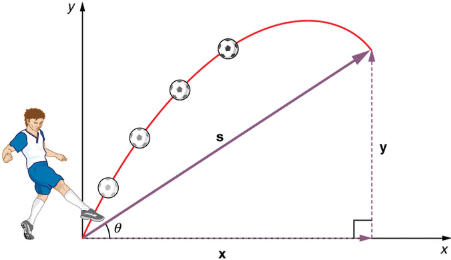Fig: Two Dimensional Motion

3. Three Dimensional Motion
If all the three coordinates specifying the position of the object changes with respect to time, then the motion is called three dimensional motion.

A few instances of three dimension are flying bird, a flying kite, a flying aeroplane, the random motion of gas molecule etc.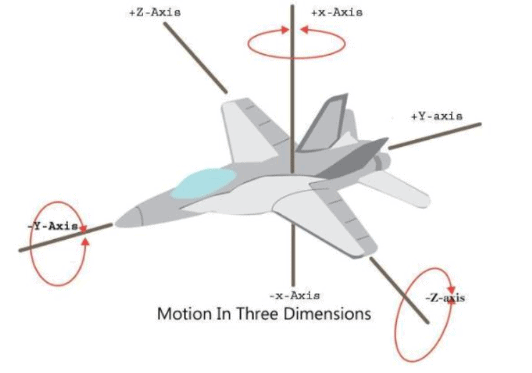Fig: Three Dimensional Motion

Distance
The length of the actual path traversed by an object is called the distance. It is a scalar quantity and it can never be zero or negative during the motion of an object. Its unit is metre.

Displacement
The shortest distance between the initial and final positions of any object during motion is called displacement. The displacement of an object in a given time can be positive, zero or negative. It is a vector quantity  and its unit is metre.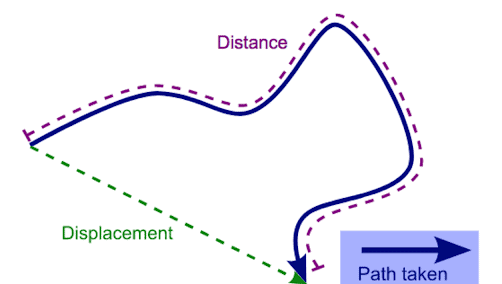Fig: Distance and Displacement

SPEED
The time rate of change of position of the object in any direction is called speed of the object.

Speed (v) = Distance traveled (s) / Time taken (t)

• Its unit is m/s.
• It is a scalar quantity.
• Its dimensional formula is [M0T-1].

Uniform Speed
If an object covers equal distances in equal intervals of time, then its speed is called uniform speed.

Non-uniform or Variable Speed
If an object covers unequal distances in equal intervals of time, then its speed is called non-uniform or variable speed.

Average Speed
The ratio of the total distance travelled by the object to the total time taken is called average speed of the object.

Average speed = Total distanced travelled / Total time taken

If a particle travels distances s1, s2, s3 , … with speeds v1, v2, v3, …, then
Average speed = s1 + s2 + s3 + ….. / (s1 / v1 + s2 / v2 + s3 / v3 + …..)

If particle travels equal distances (s1 = s2 = s) with velocities v1 and v2, then
Average speed = 2 v1 v2 / (v1 + v2)

If a particle travels with speeds v1, v2, v3, …, during time intervals t1, t2, t3,…, then,
Average speed = v1t1 + v2t2 + v3t3 +… / t1 + t2 + t3 +….

If particle travels with speeds v1, and v2 for equal time intervals, i.e., t1 = t2 = t3, then,
Average speed = v1 + v2 / 2

When a body travels equal distance with speeds V1 and V2, the average speed (v) is the harmonic mean of two speeds.
2 / v = 1 / v1 + 1 / v2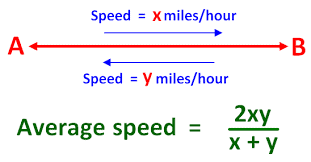Instantaneous Speed
When an object is travelling with variable speed, then its speed at a given instant of time is called its instantaneous speed.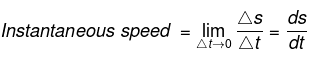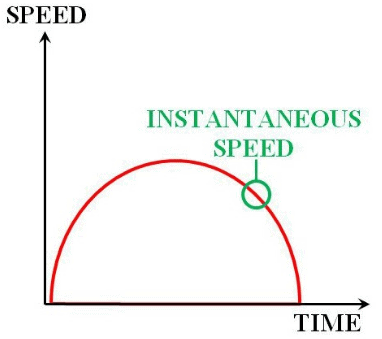Fig: Instantaneous Speed

VELOCITY

The rate of change of displacement of an object in a particular direction is called its velocity.

Velocity = Displacement / Time taken

• Its unit is m/s.
• Its dimensional formula is [MoT-1].
• It is a vector quantity, as it has both, the magnitude and direction.
• The velocity of an object can be positive, zero and negative.

Uniform Velocity
If an object undergoes equal displacements in equal intervals of time, then it is said to be moving with a uniform velocity.

Non-uniform or Variable Velocity
If an object undergoes unequal displacements in equal intervals of time, then it is said to be moving with a non-uniform or variable velocity.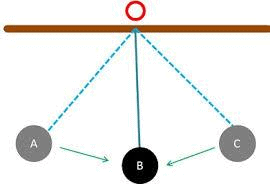Fig: Uniform Velocity

Relative Velocity
Relative velocity of one object with respect to another object is the time rate of change of relative position of one object with respect to another object.
Relative velocity of object A with respect to object B
VAB = VA – VB

When two objects are moving in the same direction, then
VAB = VA - VB
or
VAB = VA - VB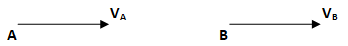When two objects are moving in opposite direction, then
VAB = VA + VB
or
VAB = VA + VB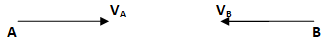When two objects are moving at an angle, then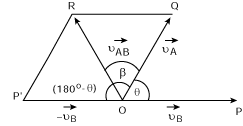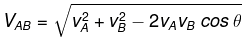and tan β = vB sin θ / vA – vB cos θ

Average Velocity
The ratio of the total displacement to the total time taken is called average velocity.
Average velocity = Total displacement / Total time taken

ACCELERATION
The time rate of change of velocity is called acceleration.

Acceleration (a) = Change in velocity (Δv) / Time interval (Δt)

• Its unit is m/s2
• Its dimensional formula is [M0LT-2].
• It is a vector quantity.

Acceleration can be positive, zero or negative. Positive acceleration means velocity increasing with time, zero acceleration means velocity is uniform while negative acceleration (retardation) means velocity is decreasing with time.

If a particle is accelerated for a time t1 with acceleration a1 and for a time t2 with acceleration a2, then average acceleration

aav = a1t1 + a2t2 / t1 + t2

DIFFERENT GRAPHS OF MOTION

1. Displacement – Time Graph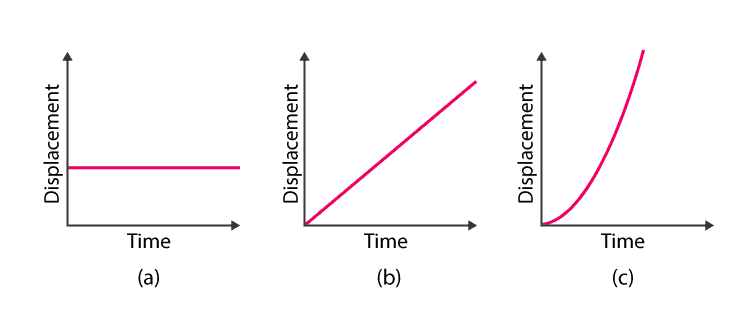Fig: Graphs for (a) Stationary Body, (b) Body moving with a Constant Velocity, (c) Body moving with a constant acceleration.

Note: Slope of displacement-time graph gives average velocity.

2. Velocity – Time Graphs: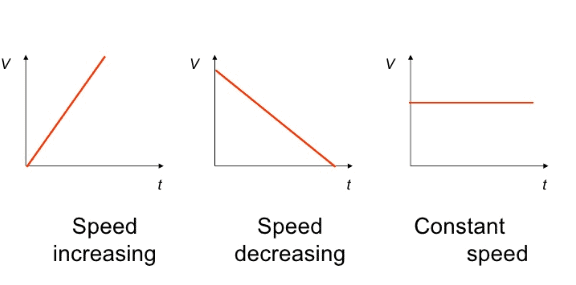Note: Slope of velocity-time graph gives average acceleration.

3. Acceleration – Time Graphs: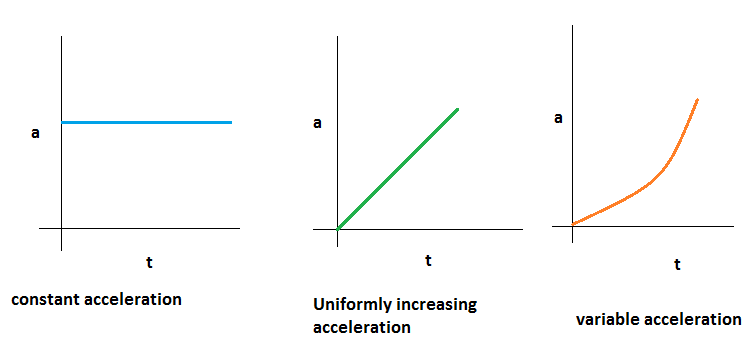Equations of Uniformly Accelerated Motion:

If a body starts with velocity (u) and after time t its velocity changes to v, if the uniform acceleration is a and the distance travelled in time t in s, then the following relations are obtained, which are called equations of uniformly accelerated motion.

(i) v = u + at
(ii) s = ut + at2
(iii) v2 = u2 + 2as
(iv) Distance travelled in nth second Sn = u + a / 2(2n – 1)

If a body moves with uniform acceleration and velocity changes from u to v in a time interval, then the velocity at the mid point of its path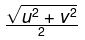Motion Under Gravity:

If an object is falling freely (u = 0) under gravity, then equations of motion
(i) v = u + gt
(ii) h = ut + gt2
(iii) V2 = u2 + 2gh

Note: If an object is thrown upward then g is replaced by – g in above three equations.
It thus follows that:
(i) Time taken to reach maximum height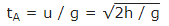(ii) Maximum height reached by the body

hmax = u2 / 2g

(iii) A ball is dropped from a building of height h and it reaches after t seconds on earth. From the same building if two ball are thrown (one upwards and other downwards) with the same velocity u and they reach the earth surface after t, and t2 seconds respectively, then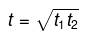(iv) When a body is dropped freely from the top of the tower and another body is projected horizontally from the same point, both will reach the ground at the same time.

SOLVED EXAMPLES
Example 1. A car travels along a straight line. For the first half it travels 20 km/h and for second half it travels 50 km/h. What is the mean speed of that car?
Solution.
In this kinematics problem we need to use definition of the average speed. The average speed is defined as the ratio of distance travelled and time travelled
vaverage = d/t....................(1)
Let us assume that the car travels distance d. Then we know that the first half, which means that distance d/2, the car travels with speed of 20 km/h. We can find the time it travels the first half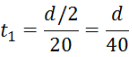problem Then the car travels the second half – distance d/2– with speed 50 km/h. We can find the time it travels this distance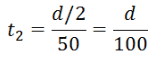Then the total time, the car travels, is the sum of t1 and t2: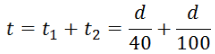We substitute this time in equation (1) and obtain the average speed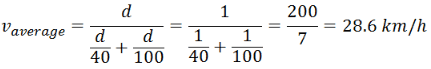Example 2. The acceleration of an object as a function of time is a(t) = -8t(m/s2) Determine the
(a) velocity and
(b) the position of the object as a function of time
if it is located at x = 2 m and has a velocity of 3 m/s at time t = 0 s.
Solution.
(a) By definition the velocity of the object is the integral of acceleration with respect to time: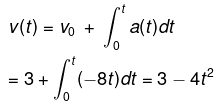where v= 3m/s is the initial velocity (at t=0).
(b) The position can found as an integral of velocity with respect to time: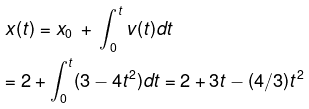where x= 2m is the initial position (at t=0) of the object.

Example 3. A rock is dropped from rest into a well.
(a) The sound of the splash is heard 4 s after the rock is released from rest.
How far below to top of the well is the surface of the water? (the speed of sound in air at ambient temperature is 336m/s).
(b) If the travel time for the sound is neglected, what % error is introduced when the depth of the well is calculated?

Solution.
(a) If h is the depth of the well then to find the time of the rock's fall we need to use the following equation: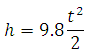From this equation we can find time: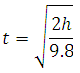After the rock hits the water the sound of splash will propagate the distance h with speed 336 m/s. After time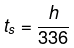the sound reaches the ground. Then total time is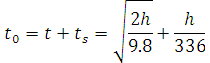This time is equal to 4 s. Then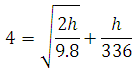From this equation we can find h:
h = 70.4m
(b) If we neglect the sound velocity then t=4 s and we can find the height from the equation: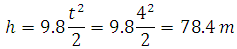If we compare this result with the result from part (a) then we can find an error: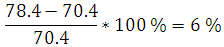Offer running on EduRev: Apply code STAYHOME200 to get INR 200 off on our premium plan EduRev Infinity!

,

,

,

,

,

,

,

,

,

,

,

,

,

,

,

,

,

,

,

,

,

;